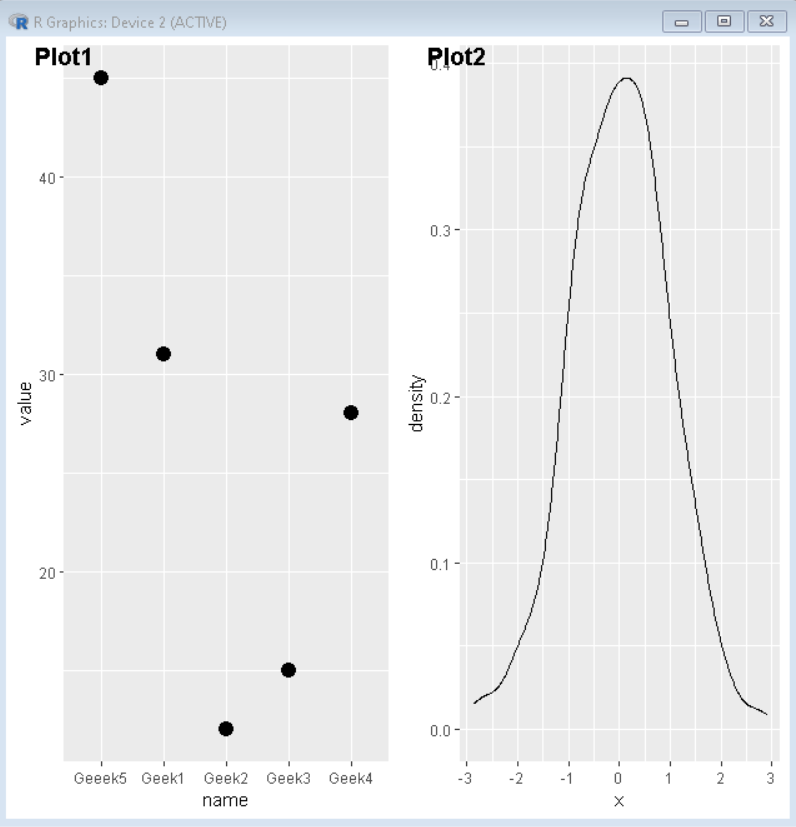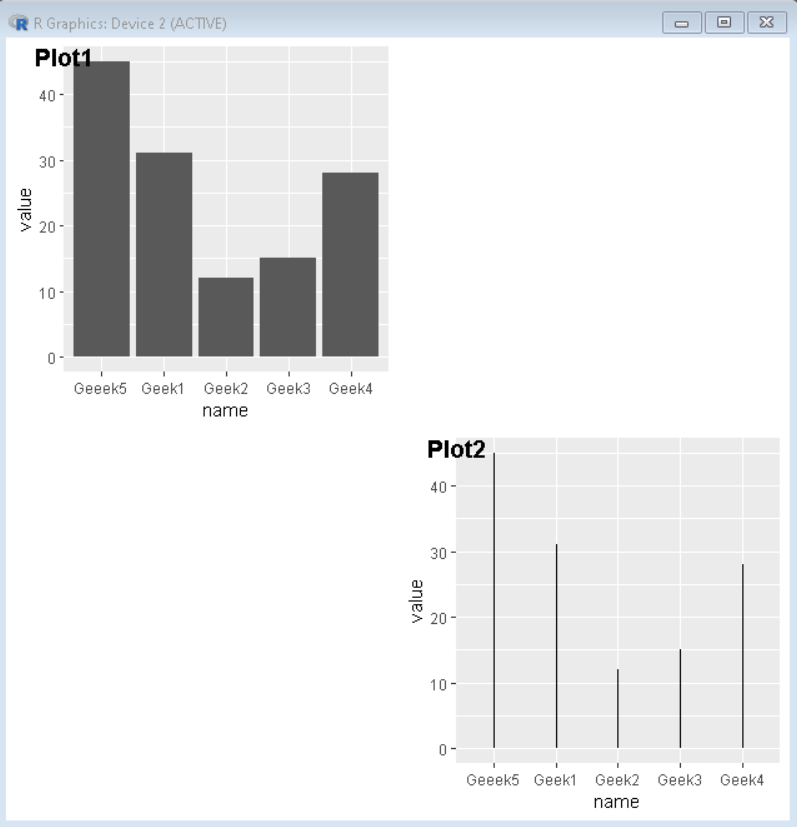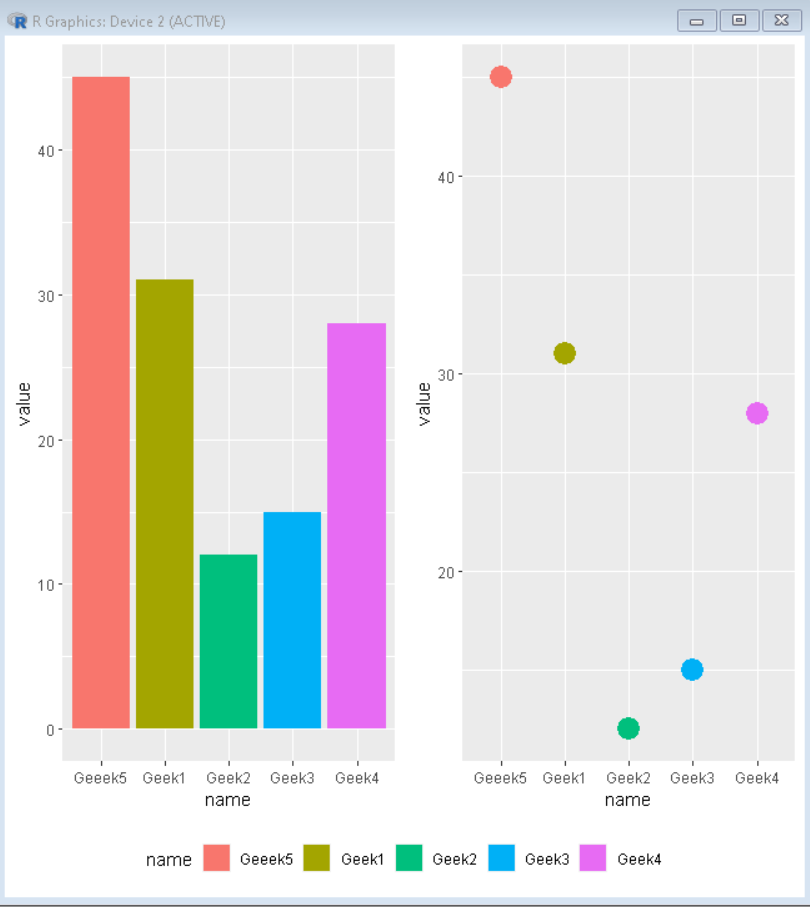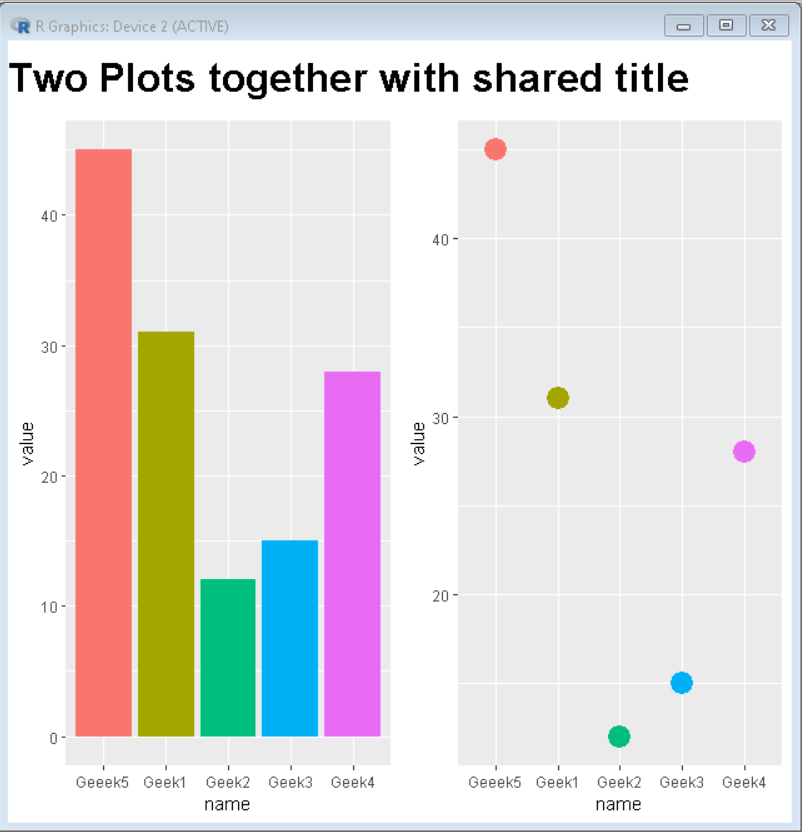How To Join Multiple ggplot2 Plots with cowplot?

• Last Updated : 17 Oct, 2021

In this article, we are going to see how to join multiple ggplot2 plots with cowplot. To join multiple ggplot2 plots, we use the plot_grid() function of the cowplot package of R Language.

Syntax: plot_grid(plot1,plot2,label=<label-vector>, ncol, nrow)

Parameters:

• plot1 and plot2 are plots that need to be joined.
• <label-vector> is a vector that has a collection of labels for plots.
• ncol is the number of columns canvas will be divided in.
• nrow is the number of rows canvas will be divided in.

R

 # Create sample dataset.seed(5642)                             sample_data1 <- data.frame(  name = c("Geek1","Geek2","Geek3",           "Geek4","Geeek5") ,  value=c(31,12,15,28,45))  sample_data2 <- data.frame(x = rnorm(400))  # Load ggplot2 and cowplotlibrary("ggplot2") library("cowplot")  # Create both plot and store in variableplot1<-ggplot(sample_data1,              aes(x = name, y = value)) +geom_point(size=4)  plot2<-ggplot(sample_data2, aes(x = x)) +geom_density(alpha=0.8)plot_grid(plot1, plot2, labels = c('Plot1', 'Plot2'))

Output:Output

Example 2: Create a gap between plots using NULL as plot value.

To fix the number of columns we use ncol attribute of plot_grid() function.

R

 # Create sample dataset.seed(5642)                             sample_data <- data.frame(  name = c("Geek1","Geek2","Geek3",           "Geek4","Geeek5") ,  value = c(31,12,15,28,45))  # Load ggplot2 and cowplotlibrary("ggplot2") library("cowplot")  # Create both plot and store in variableplot1<-ggplot(sample_data, aes(x=name, y=value)) +geom_bar(stat = "identity")  plot2<-ggplot(sample_data, aes(x = name, y=value)) +geom_segment( aes(x=name, xend=name, y=0, yend=value))   plot_grid(plot1,NULL, NULL, plot2, labels = c(  'Plot1','','', 'Plot2'), ncol=2)

Output:Output

Example 3: Side by Side plot with the shared legend.

To create a plot with a shared legend, we create plots without a legend and create a separate legend and combine them.

R

 # Create sample dataset.seed(5642)                             sample_data <- data.frame(name=c("Geek1","Geek2","Geek3",                                 "Geek4","Geeek5") ,                          value=c(31,12,15,28,45))  # Load ggplot2 and cowplotlibrary("ggplot2") library("cowplot")  # Create both plot and store in variable without legendplot1<-ggplot(sample_data, aes(x=name, y=value, fill=name)) +geom_bar(stat = "identity") +theme(legend.position = "none")  plot2<-ggplot(sample_data, aes(x = name, y=value, fill=name)) +  geom_point(aes(colour = factor(name)), size = 6)+theme(legend.position = "none")  # combine both plot using plot_grid()combined_plot<-plot_grid(plot1, plot2,ncol=2)  # extract legend from plot2legend <- get_legend(  plot1 +    guides(color = guide_legend(nrow = 1)) +    theme(legend.position = "bottom"))  # Combine combined plot and legend using plot_grid()plot_grid(combined_plot, legend,ncol=1,rel_heights = c(1, .1))

Output:Output

Example 4: Side by Side plot with a shared title

To create a plot with a shared title, we create plots without titles and create a separate title and combine them.

R

 # Create sample dataset.seed(5642)                             sample_data <- data.frame(name=c("Geek1","Geek2",                                 "Geek3","Geek4","Geeek5") ,                          value=c(31,12,15,28,45))  # Load ggplot2 and cowplotlibrary("ggplot2") library("cowplot")  # Create both plot and store in variable without legendplot1<-ggplot(sample_data, aes(x=name, y=value, fill=name)) +geom_bar(stat = "identity") +theme(legend.position = "none")  plot2<-ggplot(sample_data, aes(x = name, y=value, fill=name)) +  geom_point(aes(colour = factor(name)), size = 6)+theme(legend.position = "none")  # combine both plot using plot_grid()combined_plot<-plot_grid(plot1, plot2,ncol=2)  # create title for plottitle <- ggdraw() +   draw_label(    "Two Plots together with shared title",    fontface = 'bold',    x = 0,    hjust = 0,    size = 24,  )# Combine combined plot and title using plot_grid()plot_grid(title, combined_plot ,ncol=1,rel_heights = c(0.1, 1))

Output:Output

My Personal Notes arrow_drop_up